# Fixed point vs. Floating Point

When an analogue audio signal is converted to a digital representation, the value of the level for each sample is rounded to the nearest quantisation step (because a digital audio system does not have an infinite resolution). I’ve talked about this in detail in a past posting.

When a sample value in a digital audio stream is stored or transmitted inside a piece of audio equipment or software, one of the choices the engineer can make is whether the value should be represented using a fixed point or a floating point system. These are related, but fundamentally different, and they have some effects on the audio signal that may be audible if you’re not careful…

Let’s lay down some basic points to start. We’ll say the following:

• Audio is a kind of AC signal that has a level that can vary between two values.
• For now, we’ll say that the limits on the range of values is -1 and +1, and it can be anything in between.
• We’re going to divide up that range into some finite number of steps and round the actual signal value to the closest usable value. (I’ll assume for this posting that you already understand that dither is your friend.)
• The value will be stored as a binary number somehow

The question that we’ll look at here is exactly how that binary value represents the number, and a little of what that means to the audio signal.

## Fixed Point Representation

The simplest way to represent the value is to divide the total range from the minimum to the maximum number into an equal number of steps, and round the signal’s value to the closest step. This is a really generalised description of a “fixed point” system.

For example, if we have a 3-bit number to play with, we’ll take the first bit and use that one to represent the + or – portion of the value (where 0 means “+” and 1 means “-“). For values from 0 up to (just under) the positive maximum, the other 2 bits are used to just count the steps, from 000 up to 011. The negative values start at the bottom and work their way up to 1 step below 0, from 100 to 111. This can be seen in Figure 1.Figure 1: A simplified representation of the use of quantisation steps in a 3-bit fixed point system.

If you look carefully at Figure 1, you’ll see that there is one extra negative step, since one of the positive steps is used to represent the value 0 in the middle. This means that, if the signal is symmetrical, then we will wind up using all of the possible quantisation values except for the bottom one (just like I’ve shown in the plot), however, for the rest of this discussion, we’ll be working with numbers that are so big that this one step doesn’t really matter, so I won’t mention it again.

If we are using a 3-bit number to represent the value, then we have a total number of 23 quantisation steps: 8 of them. Each time we add one more bit, we double the number of steps. So, for a 16-bit sample, we have 216, or 65,536 possible quantisation values. For a 24-bit sample, we have 224, or 16,777,216 steps.

By increasing the number of bits in the number, we don’t change the level (it still has a range of -1 to +1), we’re just increasing the resolution that we have to make the measurement. The higher the resolution, the lower the error, and so the lower the level of distortion (if we don’t dither) or noise (if we do) relative to the signal.

If you have a fixed-point system, and you want to calculate the difference in level between the maximum signal level and the noise floor, then you can use a somewhat simplified equation, shown below:

Dynamic Range In dB ≈ 6 * nBits – 3

As I said, this is simplified due to some rounding to keep the numbers nice, but the general idea is that you have a doubling of dynamic range for every extra bit (therefore 6 dB per bit) and you lose 3 dB for the (TPDF) dither (but that’s better than not having the dither and having distortion instead). If you wanted to do it properly, then you can use this math instead:

Dynamic Range In dB ≈ 20*log10(2nBits) – 20*log10(sqrt(2))

So, if you have a 16-bit fixed point system, you have about 93 dB of range from the loudest signal to the noise floor. If you have a 24-bit system, it’s about 141 dB.

Remember that the noise floor is constant (I’m assuming it’s dithered), so as the signal level drops below maximum the current signal to noise ratio will drop by the same amount. Therefore, if your signal is 12 dB below maximum (or -12 dB FS, which means “12 decibels below Full Scale”), then the SNR in a 16-bit system is 93 – 12 = 81 dB.

If that last paragraph didn’t make complete sense, go back and read it again, because it’ll come back later…

Fixed point is a good system for conversion of an audio signal from and to analogue, but if you’re doing some really serious processing, it might not work out so well. This is due to two primary reasons:

• If your signal is going to outside the range, it will clip at the maximum positive or the minimum negative value because fixed point is not designed to exceed its range.
• If the signal is going to be reduced to a very low level somewhere in your proceeding (say, inside a biquad, for example) then you might need a LOT of bits to keep the noise floor low enough when the signal level is brought back up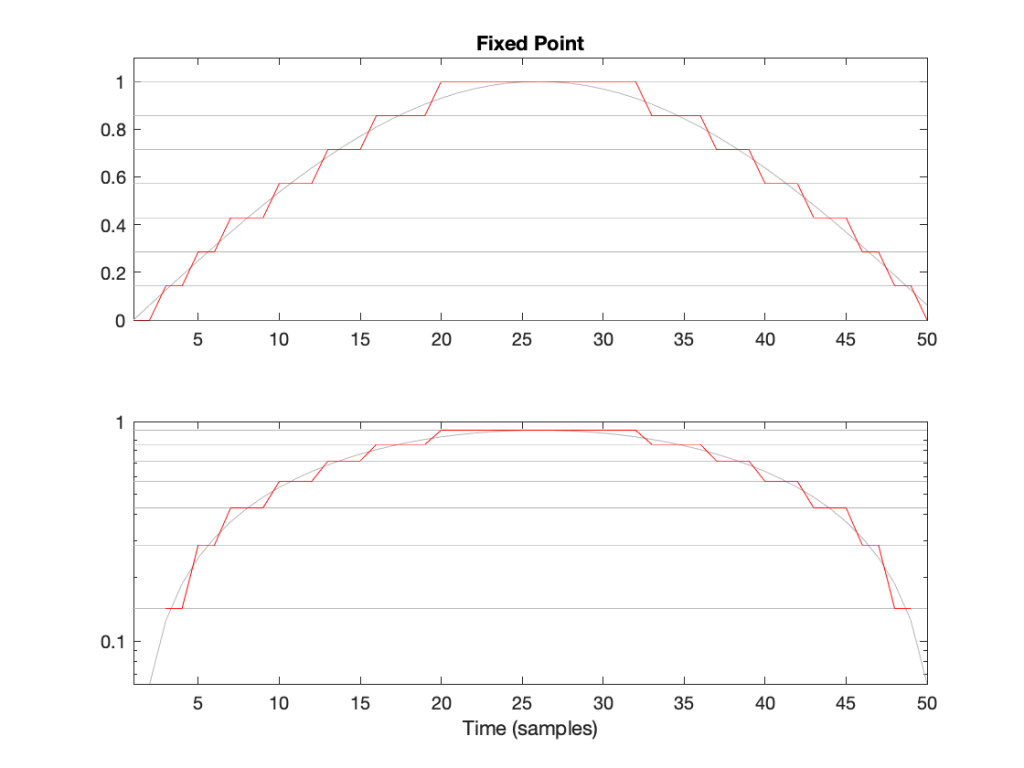Figure 2: The first half of a sine wave (in grey) quantised (without dither) in a simplified 4-bit fixed point system. (I’ve actually cheated a bit and just made 8 equally-spaced steps from 0 to 1 unlike the version shown in Figure 1.) The two plots show identical data, but the bottom plot has a logarithmically-scaled Y-axis.

As can be seen in Figure 2, the equally-spaced steps in a fixed point world mean that the quantisation error is always between -0.5 and 0.5 of a step (a “Least Significant Bit” or LSB), regardless of the level of the signal.

## Floating Point Representation

There is another way to use the bits to represent the signal value. This is to divide the binary “word” into two parts and to do a little math involving some subtraction, multiplication, and an exponent to arrive at the value. Just like in the Fixed Point case, we’ll reserve one bit for the +/- indicator.

Let’s say that we have a 32-bit value to work with. We’ll divide this up into the following:

• 23 bits for the fraction or mantissa, which we’ll abbreviate f
• 8 bits for the exponent, abbreviated e
• 1 bit for the +/- sign (just like in Fixed Point)

We’ll then do the following math:

Sample Value = ± (1 – f) * 2e

We need to know a little extra information:

• because we’re using 23 bits for f, then it can range from 0 to 223-1. In other words, stated mathematically:
0 ≤ 223*f < 223
• because we’re using 8 bits for e, then it has a total range of 28 possible values. In other words it has a range from just over -27 to just under 27. In other words, stated mathematically:
-126 ≤ e ≤ 127
(Note that a couple of possible values are reserved for special purposes, but we won’t talk about those)

This is all a little complicated, but there is a “punch line” to which I’m headed:

Unlike Fixed Point representation, the divisions of the values – the number of steps, and therefore the step sizes – are not the same across the entire scale of possible values. It’s divided into sections, where each section has quantisation steps of equal size, but that step size is dependent on what the value is. In other words the step size changes with the value, but on a coarser scale.

That step size can be calculated as follows:

From 2e to 2e+1, the steps all have an equal size of 2e-fBits where fBits is the number of bits used to express f (in the case of a 32-bit floating point word, fBits = 23 bits). In other words, we have 2fBits equally-spaced steps in that range.

Therefore, each time the signal value moves from just below 0.5 to just above (for example) then the resolution changes, and the higher the value, the lower the resolution. This is is how Floating Point representation behaves.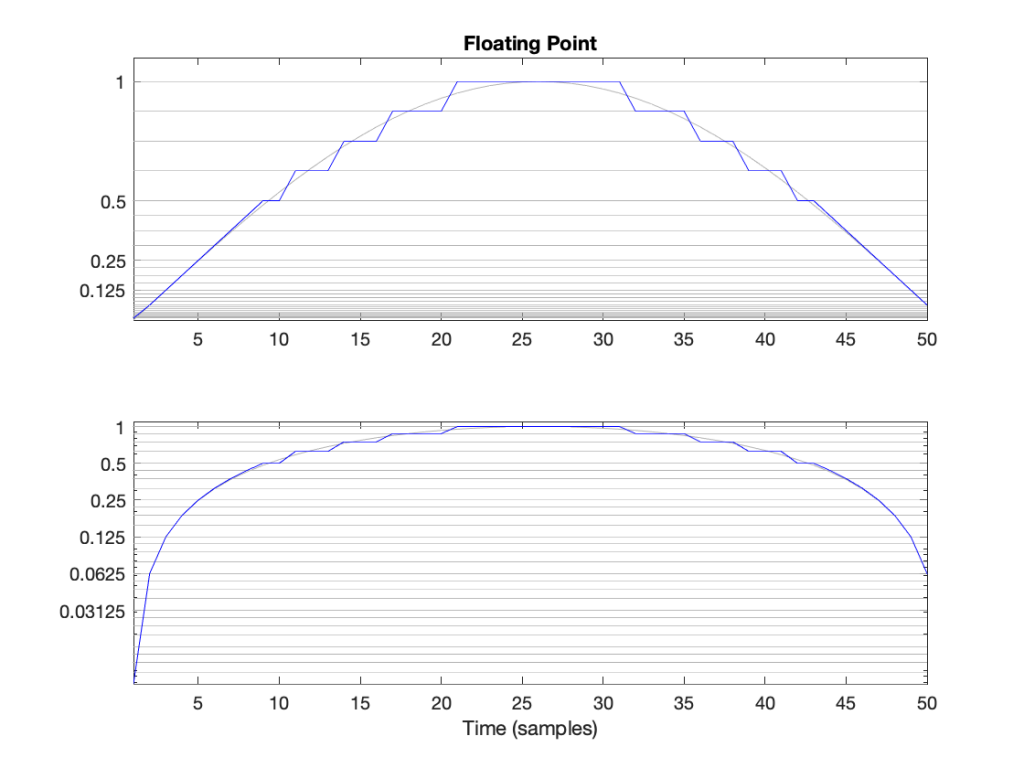Figure 3: The first half of a sine wave (in grey) quantised (without dither) in a simplified floating point system with 2 bits for the fraction. This means that there are 4 equally-spaced steps from (for example) 0.25 to 0.5 or 0.5 to 1. The two plots show identical data, but the top plot has a linearly-scaled Y-axis, whereas the bottom plot has a logarithmically-scaled Y-axis.

## Do I care?

Let’s find out.

In a 32-bit floating point world (therefore, one with a 23-bit fraction), if I have a signal that has a level that has has a maximum positive value of 1 (or 20), then the resolution of the value (which defines the error, which defines the “distance” in dB to the noise floor) is 2-25 (or 1/33,554,432).* This means that the noise floor is about 150 dB below the signal (20 * log10(1 / 2-25). As the signal level drops to 0.5, the noise floor remains the same, so the signal drops by 6 dB, and the SNR reduces to 150 – 6 = 144 dB.

Then, when we drop just below 0.5, the resolution of the value suddenly changes to 2-26 (or 1/67,108,864) , which means that the noise floor is about 150 dB below the signal (20 * log10(0.5 / 2-26). As the signal drops to 0.25 (-6 dB relative to 0.5), the noise floor remains the same, so the signal drops by 6 dB, and the SNR reduces to 150 – 6 = 144 dB.

Then, when we drop just below 0.25, the resolution of the value suddenly changes to 2-27 (or 1/134,217,728), which means that the noise floor is about 150 dB below the signal (20 * log10(0.25 / 2-27). As the signal drops to 0.25 (-6 dB relative to 0.5), the noise floor remains the same, so the signal drops by 6 dB, and the SNR reduces to 150 – 6 = 144 dB.

Hopefully, by now, you’re seeing a pattern here.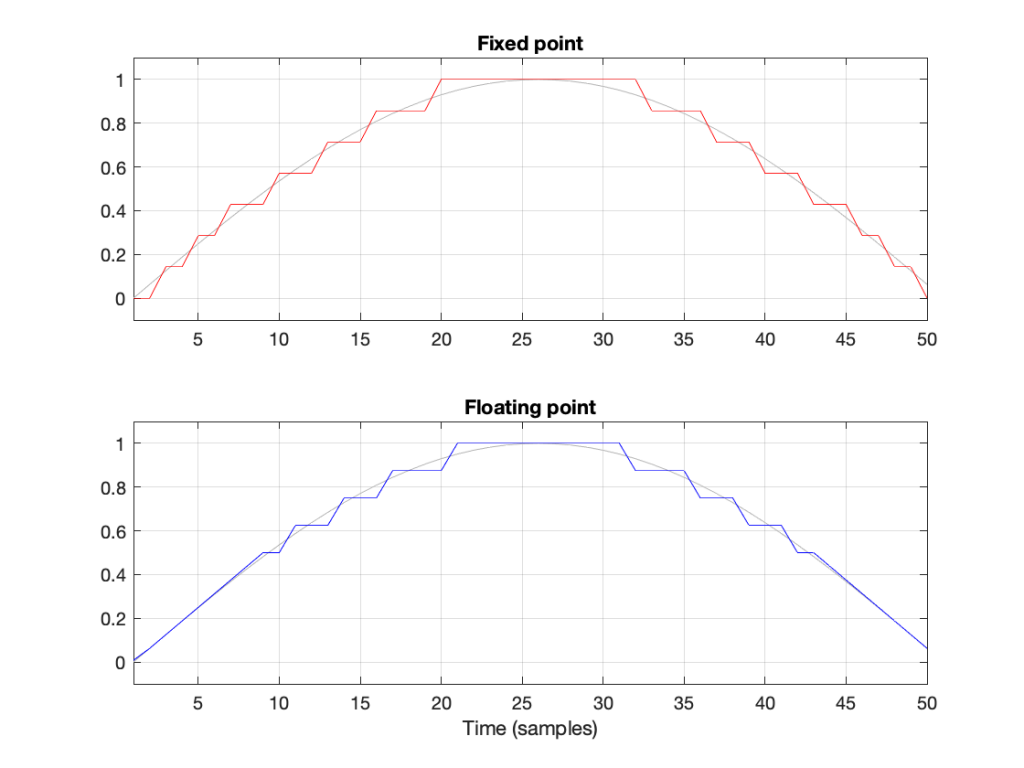Figure 4: Notice that the error of the floating point version is reduced when the signal level (in grey) approaches 0.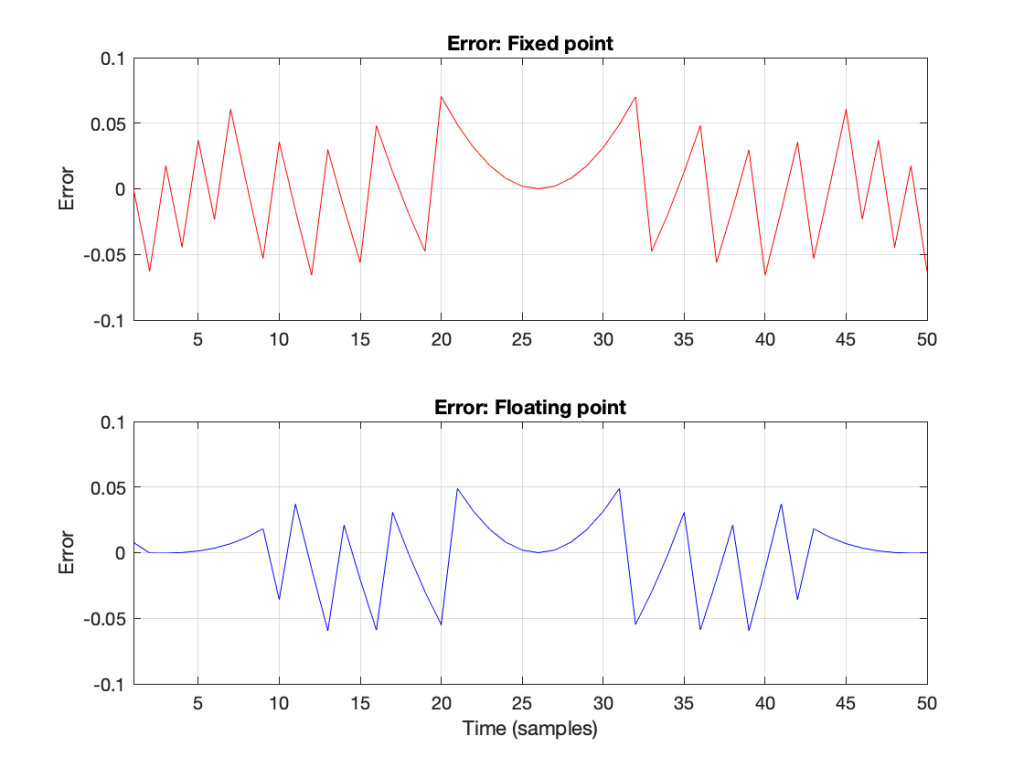Figure 6: The errors from the quantisation shown Figure 5. These are just the original signal subtracted from the quantised signals. Notice that, in Floating Point, the general level of the error is dependent on the level of the signal (it’s smaller on the left and right of the plot) whereas in Fixed Point, the overall level of the error is more constant.

The cool thing is that the pattern would have been the same if I had gone above 1 instead of below it. So, the two things to worry about in Fixed Point (inadequate resolution with (temporarily) low-level signals and clipping when the signal goes outside the range) are not problems in floating point.** And, if you have enough bits (32-bit floating point is the standard “single precision” resolution, but 64-bit “double precision” resolution is not uncommon).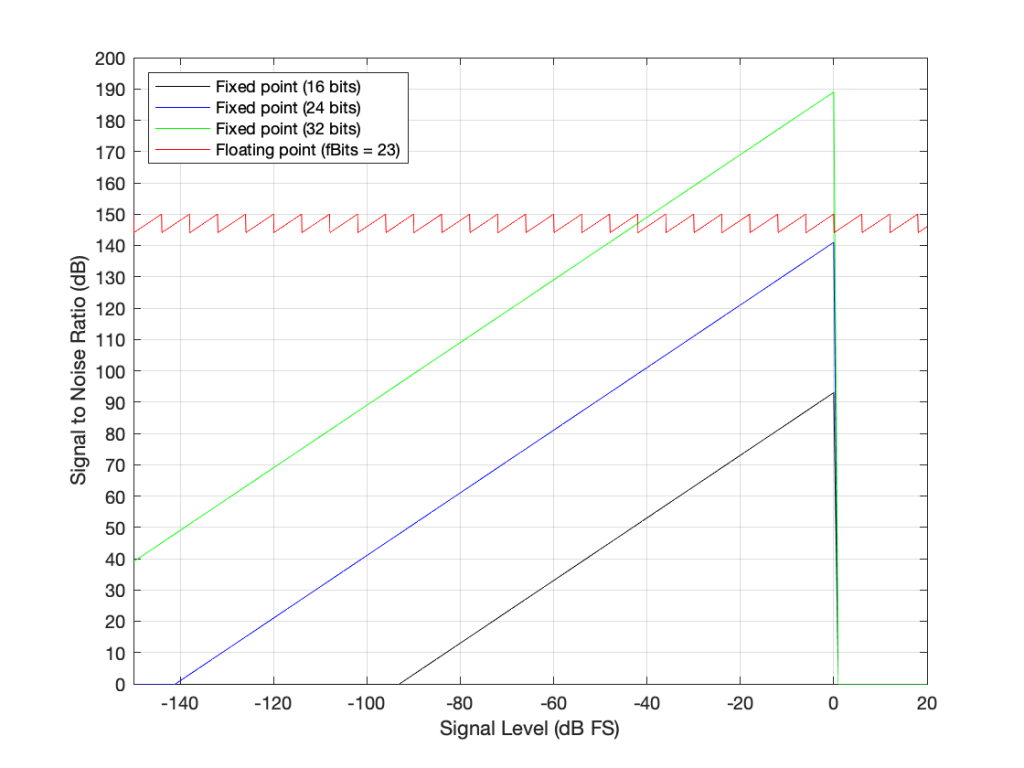Figure 7: The Signal to Distortion+Noise ratio of four different systems, as a function of the signal level in dB FS.**

This is why, in most modern audio systems, you have a fixed-point ADC and a DAC (an Analogue to Digital Converter and a Digital to Analogue converter) at the input and output of your system (because the signal range is reasonably well-defined, and the dynamic range is more than adequate if you do it right) but the processing on the inside is done in 32-bit or 64-bit floating point (or both, in some devices) so that the engineers have the resolution and the range to play with the signals before getting them ready for the output.***

There may be some argument made for a constant noise floor level in a fixed-point system (assuming it’s dithered) over a signal-modulated noise level in a floating-point world (assuming it’s not), however, there are two reasons why this is likely not a real-world issue. The first is that, even in a single-precision floating point system, the worst-case signal to noise ratio is about 144 dB, which is very good. The second is that smart people have already been thinking about dither for floating point systems. If this sounds interesting, you can start reading here

## One last thing

You may be wondering about that sawtooth plot: the red line in Figure 7. It can’t keep going forever, right?

Right.

Eventually, if the signal is quiet enough, then you run out of exponents and the system just behaves as a 23-bit fixed point system (assuming a 32-bit floating point). This will happen when e = -126. Below that, then the SNR just follows a downward slope just like the fixed-point plots. If the signal is loud enough (when e = 127) then you’ll clip, again, just like the fixed-point systems do when the input signal has a level of 0 dB FS.

So, then the question is: “how quiet / loud does the input signal have to be for that to happen?” The answer is very quiet and very loud, as you can see in the plot in Figure 8.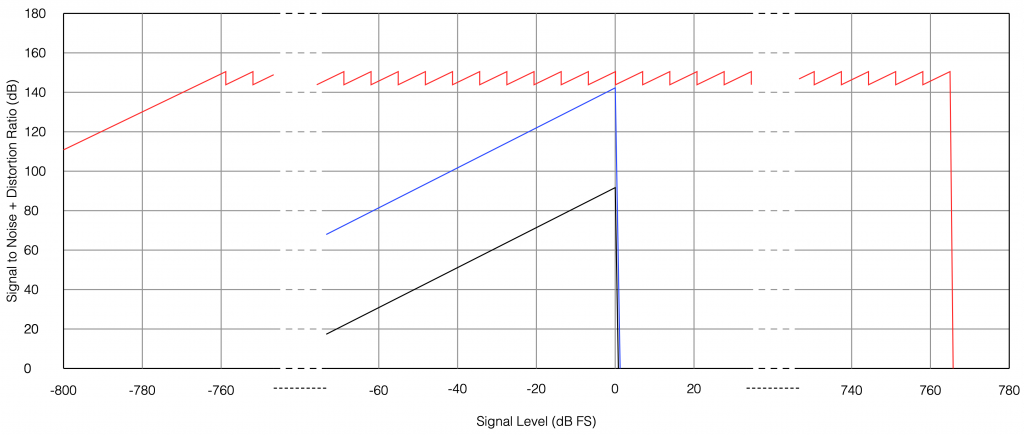Fig 8. The limits of a 32-bit floating point signal. As you can see, you’ve got plenty of dynamic range to work with before you run out of room on either side. The black line is 16-bit fixed point, the blue line is 24-bit fixed point, and the red line is 32-bit floating-point.

You may be wondering how I calculated those limits:

• The first peak in the sawtooth on the left side is at 20*log10(2^-126) = -758.6 dB FS
• The last peak in the sawtooth on the right side is at 20*log10(2^127) = 764.6 dB FS
• The slope that just below the 0 dB FS Signal level is where e = -1. The slope just above 0 dB FS is where e = 0.

## * First small note for the attentive

You may have noticed what appears to be a mistake in my math in there. First I said:

From 2e to 2e+1, the steps all have an equal size of 2e-fBits where fBits is the number of bits used to express f (in our case, fBits = 23 bits). In other words, we have 2fBits equally-spaced steps in that range.

Then I did the math and said

In a 32-bit floating point world (therefore, one with a 23-bit fraction), if I have a signal that has level that has just come up to 1 (or 20), then the resolution of the value (which defines the error, which defines the “distance” in dB to the noise floor) is 2-25 (or 1/133,554,432).

Why did I say 2-25 when maybe I should have said 2-23 (because there are 23 bits in the fraction)? The reason is that the 223 quantisation levels are located between 1 down to 0.5. If I were to continue with the same spacing down to 0, then I would have twice as many quantisation levels, so there would be 224 instead. If I were to continue the spacing all the way down to -1, then there would be twice as many again, or 225.

In other words, a floating point signal ranging from a value of 2-1 to 20 (0.5 to 1) with some number of bits in the fraction that we’re calling fBits will have almost exactly the same signal to noise ratio as an non-dithered fixed point system that is scaled to range from -1 to 1 with fBits+2.

This would be the same from -20 to -2-1 (-1 to -0.5).

At any other signal value, the quantisation behaviours (and therefore the signal-to-noise ratios) of the two systems will be significantly different.

This is visible in Figure 6 where, when the signal is high (in the middle of the plots), the error level is approximately the same in the 4-bit fixed-point system and the floating point system with 2 bits for the fraction.

## ** Second small note for the attentive

You will notice that the black, blue, and green lines in Figure 7 have a sharp transition when the signal level hits 0 dB FS. This is because, in a fixed point system at signal levels below 0 dB FS, the signal to noise ratio is the difference in level between the dither’s noise floor and the signal. The dither level is constant, so as the signal level increases, it gets “further away” from the noise floor until you reach 0 dB FS (with a sine wave), as which point you reach the maximum possible SNR. However, once the signal goes beyond 0 dB FS (still assuming it’s a sine wave), then it starts to clip and distortion components are generated. It does not take much increase in level to drastically increase the level of the distortion relative to the level of the signal (since the signal level cannot increase – you’re just increasing distortion artefacts). Consequently, the signal to distortion+noise drops dramatically, because the distortion components increase in level dramatically.

This does not happen with the floating point system because, at 0 dB FS, you just change the exponent and keep going up with the signal level until you reach the maximum possible exponent value, which goes far beyond what I’ve plotted here.

## Third small note for the attentive

You may be looking at Figure 7 and wondering why the fixed point plots and the floating point plots don’t overlap anywhere. For example, look where the green line (32-bit fixed point) crosses the red line (32-bit floating point). Why don’t they overlap each other there for that little 6 dB-wide range on the X-axis?

The reason is that I’m modelling the fixed point SNRs with TPDF dither, which “costs” 3 dB, but I’m assuming that the floating point signal is not dithered (which would normally be the case). If I were pretending that fixed point didn’t include the dither, then the plots would, indeed, overlap each other for that narrow little window.

## ***One last comment

You may be saying to yourself “But this is nonsense! Why do I need 150 dB SNR when the signal level is lower than -100 dB FS?” The long answer is in this posting, but the short answer is that the signal can go VERY low and VERY high inside a filter (a biquad), so you need to worry about this if you’re doing any changes to the magnitude response of the signal, for example…